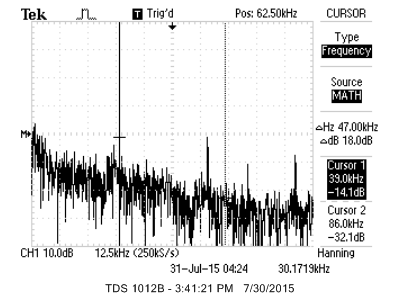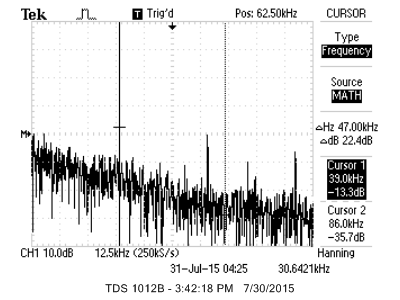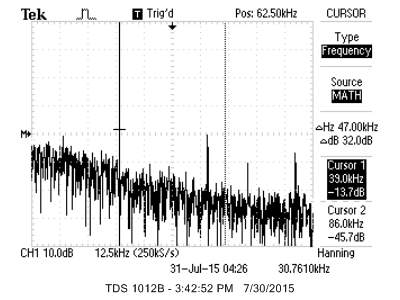Cornell University ECE4760
Random number generation

PIC32MX250F128B

Random Numbers
Generating noise is useful in games, simulation and audio. Making a repeatable sequence of pseudorandom numbers is easy using the `stdlib.h` function `rand()`. For a given seed, set using `srand(seed)`, the sequence is always the same, but sequential numbers generated are relatively uncorrelated. But see the wiki article for much more information. There are other approaches to pseudornamdom generation, including linear-feedback shift registers, which are very easy to implement and quick to execute. But what if you want to get a different sequence every time? Then you need a random seed. No process which is phase-locked to the system clock can give you a random seed. You need some source of unsynced entropy! The source could be a user generated button push interval or an ethernet packet arrival time. In these examples, I used a floating ADC input.

1. In the first example, I used an unterminated (floating) ADC input as a source of entropy, followed by a second, shifted, ADC reading 1 millsecond later, then use that 15 bit number as a seed for the `rand()` function. The images below show hstograms of 15-bit values, binned into 240 bins. The left image uses the full algorithm, the right image is just the shifted sum of the two ADC readings. Both histograms are fairly flat. With about 24,000 points in 240 bins, there is an average of 100/bin, so the RMS noise on the histogram should be about 10%. (project ZIP)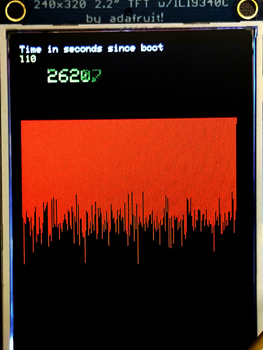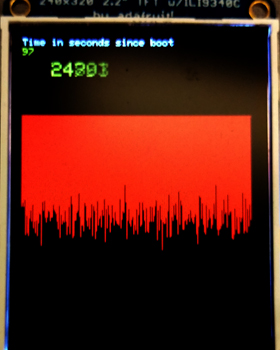2. In the second example, bandlimited white noise is generated on a PWM channel using a new random seed every time you ask for a new seed. There is an ISR running at 39062 Hz (40 MHz/1024) which generates the white noise using `rand()`, does the filtering, and sets to the 10-bit PWM sample rate. OC3 is used and is connected to pin 21 using the RPB10 in PPS. There are three threads, a keypad thread, a system timer thread, and the seed generator thread.
-- Spawns a seed thread to get a new seed, and resets the histogram, when the # key is pressed
-- Turns off a PWM random noise generator when 0 key is pressed
-- Turns on a PWM random noise generator and plots a histogram of noise when 1 key is pressed
-- Sets a filter coefficient when keys 1 to 7 are pressed. Frequencies vary from 0.005 to 0.5 of the Nyquist frequency.
The code uses 2.14 fixed point for the digital lowpass filter. (project ZIP).

The left image below shows the power spectrum when the digital fiter is set to 1KHz and filtered through an analog RC lowpass with a cutoff frequency of 10,000 radians/sec. Spectrum is flat up to about 1 KHz, then drops 10 or 12 db over the next factor of two in frequency, correspoinding to a 2-pole filter (one digital, one analog). Higher frequency spikes are caused by aliasing of the PWM carrier frequency.

The right image below removes the analog filter and sets the digital filter to 9.8 KHz. You can see a clear dropoff at about 9 or 10 KHz, bottoming out at 21 KHz, then increasing again with a large spike at the location of Cursor-1, which is at 39 KHz, the PWM carrier frequency. My guess is that the secondary hump and all the other spikes are aliased data because of the energy at the PWM carrier frequency. Since human hearing stops around 20 KHz, the high frequency junk is inaudible. Of course outputing the data to a real DAC would remove much of the high frequency, aliased, energy.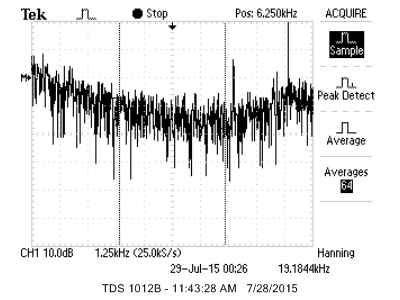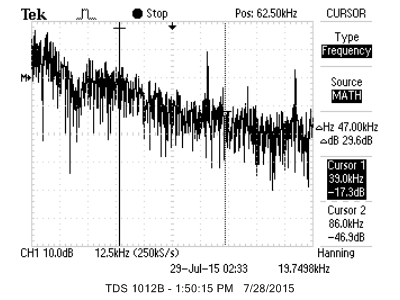3. This example generates noise using a linear feedback shift register (LFSR) instead of `rand()`. The four statements makeing one shift operation take 10 cycles to execute, compared to 35 cycles for rand(). As in the last example, bandlimited white noise is generated on a PWM channel using a new random seed every time you ask for a new seed. There is an ISR running at 39062 Hz (40 MHz/1024) which generates the white noise using the LFSR, sets the number of LFSR shifts/sample, and sets to the 10-bit PWM sample rate. There are three threads, a keypad thread, a system timer thread, and the seed generator thread. (project ZIP)